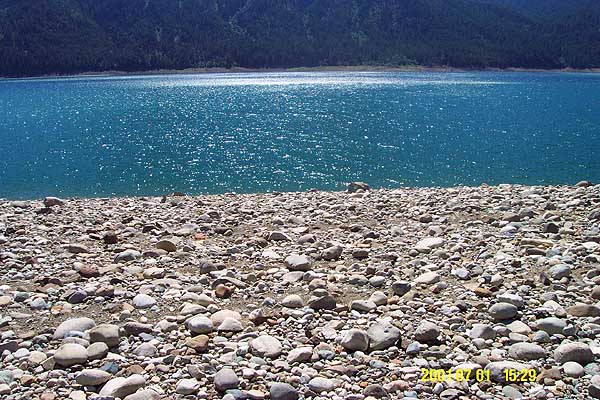Counting Rocks at Wish Poosh

By Dan Allen
Q: How many rocks were on the beach on the west side of Picnic Island?
A: A lot.  We set out to make a good "back of the envelope" estimate.

Q: How did we calculate it?
A: We determined the number of rocks in a small area and
extrapolated to the beach area.

Q: How many rocks 4 inches or larger were in a 3 ft x 3 ft area?
A: 123 rocks, determined by manual counting.

Q: How big was the beach area?
A: It was large enough that we used a GPS that we had with us to measure it.

Q: What kind of GPS?
A: A Garmin GPS III.

Q: How accurate was it?
A: Tracking 6 satellites it had roughly 13 foot accuracy.

Q: Where were the corners of the area?
A: NW corner: N 47° 16.708'  W 121° 5.635'
A: NE corner: N 47° 16.721'  W 121° 5.614'
A: SW corner: N 47° 16.654'  W 121° 5.607'   2216 ft ASL
A: SE corner: N 47° 16.662'  W 121° 5.581'   2246 ft ASL

Q: So how big was the beach area?
A: We plugged the corner coordinates into a formula to find out.

Q: What formula?
A: The great circle distance formula:
Dist = ArcCos(Sin(Lat1)*Sin(Lat2)+Cos(Lat1)*Cos(Lat2)*Cos(Lon1-Lon2))
Distance in feet = Dist*60*1852/.3048

Q: So how wide was the beach area?
A: At the north end, 102 feet.

Q: So how long was the beach area?
A: 302 feet along the shore.

Q: Was it a perfect rectangle?
A: No.  It was 205 feet wide at the south end.

Q: So it was more of a trapezoid?
A: Well yes, as the length up where we ate lunch was 385 feet long.

Q: So what did it look like?
A: A bit like Idaho.

+-- 102' --+
|          |
|          |
|          \
|           |
|           \
|            |
|             \
302'            |
|             385'
|               \
|                |
|                 \
|                  |
|                   \
|                    |
+------- 205' -------+

Q: So what shape is it?
A: As drawn it appears to be a trapezoid.

Q: So how do you figure the area of a trapezoid?
A: As the area of a rectangle plus the area of a triangle.

Q: What is the rectangle?
A: 302 feet by 102 feet = 30,804 square feet.

Q: What is the area of the remaining right triangle?
A: Area = base * height / 2
or
(205 feet - 102 feet) * 302 feet / 2 = 15,553 square feet.

Q: What is the total area of the trapezoid?
A: 30,804 + 15,553 = 46,357 square feet.

Q: Hmm.  What if none of the sides were actually parallel?
A: This is quite likely.  It was very rocky and I am quite
sure that I wandered a bit.

Q: What would this do to our area of a trapezoid calculation?
A: It would make it wrong.

Q: What do we do then?
A: We should treat our area as an arbitrary polygon.

Q: Is that different than a regular polygon?
A: Yes.  An arbitrary polygon has sides of different lengths.

Q: What is the formula for the area of just such a polygon?
A: sum += (x[i+1] - x[i]) * (y[i+1] + y[i])
with i := 1 .. n for the number of points
with x and y being the coordinates of each point
Then, the final Area = Abs(sum/2)

Q: What is the total area of the polygon using this formula?
A: 54,763 square feet.

Q: How much larger is this than if it were a trapezoid?
A: 18% larger.

Q: So how many rocks were there?
A: 54763 ft^2 / 9 ft^2/yd^2 * 123 rocks/yd^2 = 748,428 rocks

Q: That's a lot of rocks.
A: That wasn't a question, but you're right!

A: Well, it depends on every square yard containing the same number of
rocks, and we could see that some places had several large boulders
instead of lots of smaller rocks, so this assumption is certainly
false.

Q: How could you improve your estimate?
A: By measuring other 3 foot squares and taking an average, or just
counting a larger area, by using a ruler to be more exact, etc.

Q: Are you going to do that?
A: No.  This was just for fun and it bored the kids to death.
I, however, got a lot of joy out of it.

Q: Why?
A: I don't know.  I just did.  Perhaps it was because I could
visualize the population of South Dakota.

Q: How could a bunch of rocks do that?
A: Well in the 2000 Census, South Dakota had 754,844 people.
If each rock was one person, I was looking at 99.2% of
the population of South Dakota.

Q: What if you wanted to visualize a city instead?
A: San Francisco California had a Year 2000 Census total of 776,773 people.
San Francisco has 3% more people that this hill had rocks.
Probably tourists visiting!

Q: Well, I'm tired of counting rocks.
A: Me too.  Time to count gas stations...